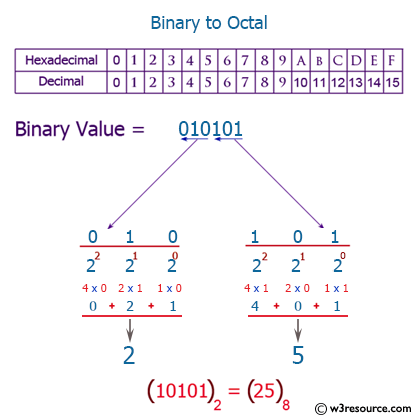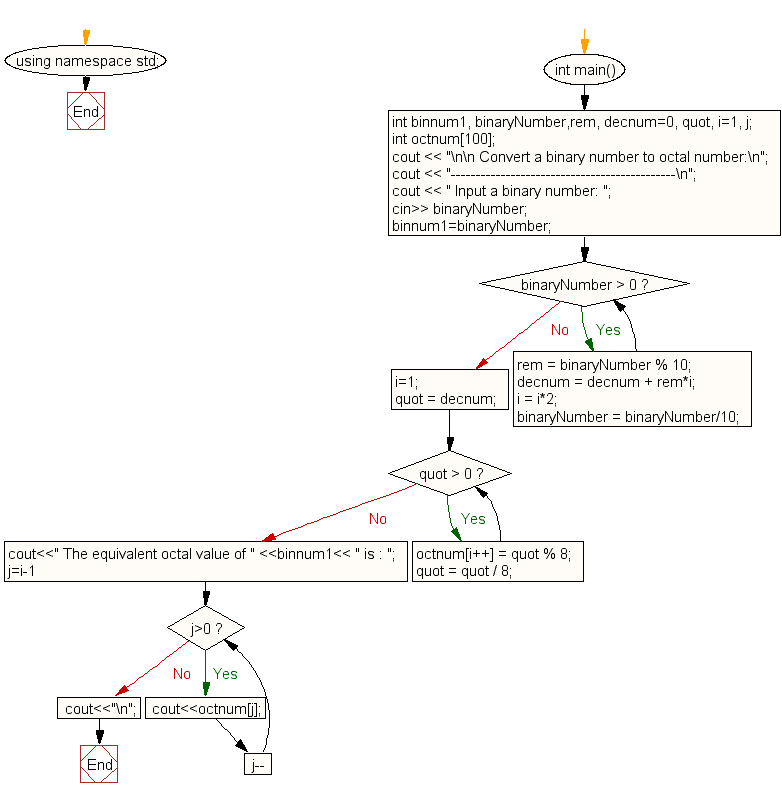﻿ C++ Exercises: Convert a binary number to octal number - w3resource

# C++ Exercises: Convert a binary number to octal number

## C++ For Loop: Exercise-75 with Solution

Write a C++ program to convert a binary number to an octal number.

Pictorial Presentation:Sample Solution:-

C++ Code :

``````#include <iostream>
#include <math.h>
using namespace std;

int main()
{
int binnum1, binaryNumber,rem, decnum=0, quot, i=1, j;
int octnum;
cout << "\n\n Convert a binary number to octal number:\n";
cout << "---------------------------------------------\n";
cout << " Input a binary number: ";
cin>> binaryNumber;
binnum1=binaryNumber;
while(binaryNumber > 0)
{
rem = binaryNumber % 10;
decnum = decnum + rem*i;
i = i*2;
binaryNumber = binaryNumber/10;
}
i=1;
quot = decnum;

while(quot > 0)
{
octnum[i++] = quot % 8;
quot = quot / 8;
}
cout<<" The equivalent octal value of " <<binnum1<< " is : ";
for(j=i-1; j>0; j--)
{
cout<<octnum[j];
}
cout<<"\n";
}
``````

Sample Output:

``` Convert a binary number to octal number:
---------------------------------------------
Input a binary number: 1011
The equivalent octal value of 1011 is : 13
```

Flowchart:C++ Code Editor:

What is the difficulty level of this exercise?

﻿

## C++ Programming: Tips of the Day

Using C++ library in C code

You will need to write an interface layer in C++ that declares functions with extern "C":

```extern "C" int foo(char *bar)
{
returnrealFoo(std::string(bar));
}
```

Then, you will call foo() from your C module, which will pass the call on to the realFoo() function which is implemented in C++.

If you need to expose a full C++ class with data members and methods, then you may need to do more work than this simple function example.

Ref: https://bit.ly/3clHy43

We are closing our Disqus commenting system for some maintenanace issues. You may write to us at reach[at]yahoo[dot]com or visit us at Facebook